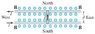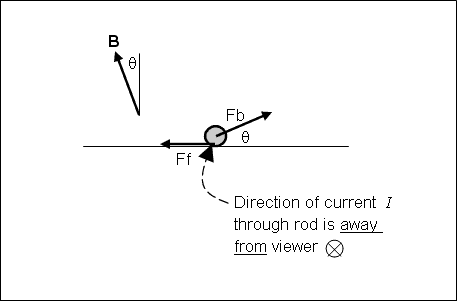# Magnetism problem for General Physics 2

• Jscrub
F=ma...to the equation for F, you get the following:F=ma (μs·FN)+frictionwhere friction is the force opposing the motion of the rod.

#### Jscrub

Magnetism problem for General Physics 2!

## Homework Statement

Suppose the rod in the figure has mass m= 0.40kg and length 20cm and the current through it is I= 40A .
(Intro 1 figure)

If the coefficient of static friction is us= 0.55, determine the minimum magnetic field (not necessarily vertical) that will just cause the rod to slide. Give the magnitude of B.

## Homework Equations

I am not really sure what equations I am supposed to use for this problem..I don't really know how to get started...can someone help me please!

I think F=ma...using the static coeff. us=.55
Maybe..F=qv x B
or F = qvB sin (theta)

## The Attempt at a Solution

#### Attachments

•GIANCOLI_ch27_p59_60.jpg
7.2 KB · Views: 415

I'd start by drawing a free body diagram for the rod. You'll need to think of all the forces that act on the rod.

F=qv x B

Hmmm, I don't think that will help here. Magnetism problems usually contain one of the following two scenarios:
• A charge q moving with velocity v, or
• A wire carrying a current I
Here we are dealing with the 2nd type, so look for an equation dealing with I, not q & v.

More hints: they have given us the mass and the friction coefficient. What forces would arise from that information?

The only equation I have with I:

F = ILB sin (theta)

But they are 90 degrees from one another so wouldn't it be Fmax = ILB

Good, that's the right equation. And yes, θ=90o here.

In this situation would sumF =mg-Fn...however I know that I would have to incorporate the us coeff. , which is related to Fn if I remember from Physics I...

not sure how to draw the free body diagram for this picture...

Jscrub said:
In this situation would sumF =mg-Fn...however I know that I would have to incorporate the us coeff. , which is related to Fn if I remember from Physics I...
Friction force Ff is
Ff = μs FN

not sure how to draw the free body diagram for this picture...
Draw the rod first, you could even just represent it as a point. Then draw vectors (arrows) to represent each force that is present, in the appropriate direction. For example, the rod's weight would be drawn as:

Code:
  o
|
|
\|/ mg

I understand how to do free force diagram..I just don't understand how this one is supposed to be...is the fn force coming from the other rod?? When it talks about the rod sliding...what is it sliding on?

I figured...

F=ma, FN=mg, friction=usFN, which means friction = (us)mg

friction is pushing against the force moving it...

I ended up with f=mgus...plugged it into

F=ILB,

Or
B=Fmax/IL...I got O.22/(40A)(.2m) =.0275T...which is not right...can someone help me please!

Jscrub said:
I understand how to do free force diagram..I just don't understand how this one is supposed to be...is the fn force coming from the other rod?? When it talks about the rod sliding...what is it sliding on?
The rod is sliding along two rails, oriented East-West as shown in the figure you posted. That is where the friction comes from.

Jscrub said:
I figured...

F=ma, FN=mg, friction=usFN, which means friction = (us)mg

friction is pushing against the force moving it...

I ended up with f=mgus...plugged it into

F=ILB,

Or
B=Fmax/IL...I got O.22/(40A)(.2m) =.0275T...which is not right...can someone help me please!
You're method would be correct if B were vertical and that magnetic force FB were horizontal.

Since the problem statement said B is not necessarily vertical, then FB need not be horizontal. FB can have a vertical component, which enters into the expression for FN:

FN + FB-vertical = mg

the equation does not incorportate friction...

FN + FB-vertical = mg
__________________

This equation says FB and FN are in the same direction...which means mg and friction are in the same direction..I don't understand how this works...

If the force is pushing it to the left or right, wouldn't friction and Fb be to the left and right

Redbelly98 said:
FN + FB-vertical = mg

Jscrub said:
the equation does not incorportate friction...
That's correct. The equation I wrote is for the vertical forces only. Friction, being a horizontal force, should not appear, but is still equal to μs·FN.

Jscrub said:
FN + FB-vertical = mg
__________________

This equation says FB and FN are in the same direction
No. FB-vertical is the vertical component of FB. But FB itself is neither horizontal nor vertical.

...which means mg and friction are in the same direction..I don't understand how this works...

If the force is pushing it to the left or right, wouldn't friction and Fb be to the left and right

It sounds like you have not yet drawn a correct free-body diagram to help visualize what is going on. Here is one showing two of the four forces involved, as well as the direction of B:Side view, showing the rod viewed end-on​

If you add mg and FN to the figure (both are vertical forces), you can then set the net force in the vertical and horizontal directions equal to zero, and go from there.

I got:

Fn=mg-I*l*b*cos(theta)?

So that I*l*b*cos(theta)=(mg-I*l*b*sin(theta))*.55?

Or did I mess up my expression for Fn?
I don't really know what to do from here...

CAN ANYONE HELP WITH THIS PROBLEM PLEASE...IT IS DUE IN 2 HOURS...

Last edited:

I got B...but I don't know how to figure out theta?help!

Nevermind...I got it